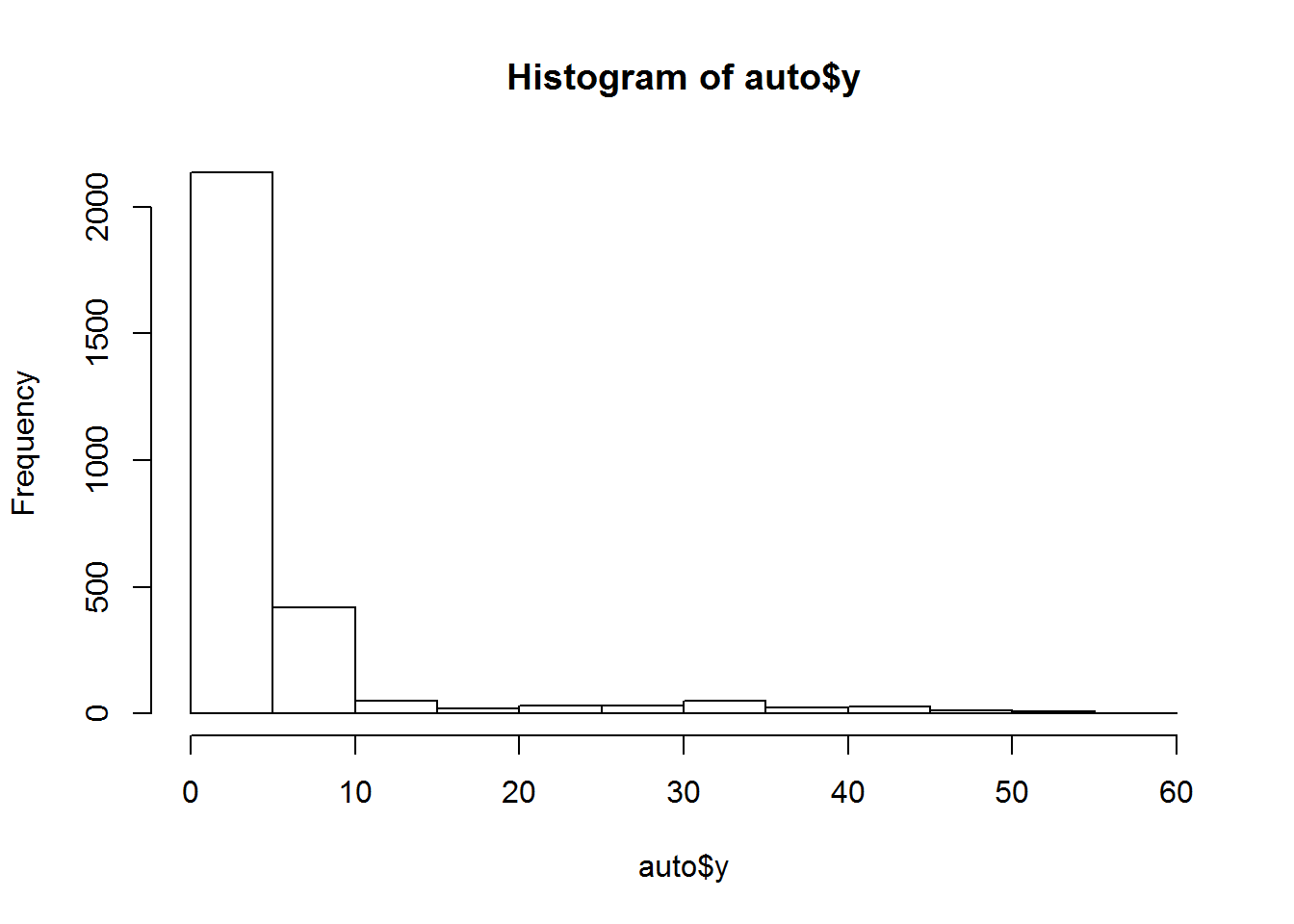## Introduction to Gradient Boosting (Generalized Boosting Model)

Gradient boosting is a machine learning technique for regression and classification problems, which produces a prediction model in the form of an ensemble of weak prediction models, typically decision trees. It builds the model in a stage-wise fashion like other boosting methods do, and it generalizes them by allowing optimization of an arbitrary differentiable loss function.

A natural regularization parameter is the number of gradient boosting iterations M (i.e. the number of trees in the model when the base learner is a decision tree). Increasing M reduces the error on training set, but setting it too high may lead to overfitting. An optimal value of M is often selected by monitoring prediction error on a separate validation data set. Besides controlling M, several other regularization techniques are used.

## xgboost package notes

This R package is an interface to Extreme Gradient Boosting, which is a implemntation to the gradient boosting framework.

library(xgboost)
library(HDtweedie) # has example dataset
data("auto")

hist(auto$y) # tweedie distribution### create a test and train subset using caret library(dplyr) auto2 = tbl_df(as.data.frame(auto)) # create a split sample; alternative method #sub <- sample(nrow(auto2), floor(nrow(auto2) * 0.66)) #train_auto <- auto2[sub, ] # ~66% #test_auto <- auto2[-sub, ] # ~33% # create a split based on the outcome of y which preserves the response distribution library(caret) set.seed(3456) trainIndex <- createDataPartition(auto2$y, p = .66,
list = FALSE,
times = 1)
head(trainIndex)
##      Resample1
## [1,]         1
## [2,]         2
## [3,]         3
## [4,]         4
## [5,]         6
## [6,]         7
train_auto <- auto2[trainIndex, ]
dim(train_auto)
##  1857   57
test_auto <- auto2[-trainIndex, ]
dim(test_auto)
##  955  57

### turn auto2 back into a list for xgboost

# train
lab_train = as.list(train_auto$y) dat_train = as.matrix(train_auto[, -1]) # test lab_test = as.list(test_auto$y)
dat_test = as.matrix(test_auto[, -1])

### model training

Since this package does not account for a tweedie distribution, the RMSE is considerably high for this dataset.

bst <- xgboost(data = dat_train, label = lab_train,
max.depth = 2, eta = 1, nthread = 2,
nround = 2, verbose = 2, objective = "reg:linear")
## tree prunning end, 1 roots, 6 extra nodes, 0 pruned nodes ,max_depth=2
##   train-rmse:7.820714
## tree prunning end, 1 roots, 6 extra nodes, 0 pruned nodes ,max_depth=2
##   train-rmse:7.735143

### model prediction / scoring

pred <- predict(bst, dat_test)
# the size of the prediction vector (955 for the amount of rows in the test data)
print(length(pred))
##  955
# print the first 10 predictions
head(pred, n=10)
##    1.6216886  3.2809963  1.6216886  1.6216886  0.4075823  1.6216886
##    0.4075823  1.6216886  1.6216886 10.1385689

### measuring model performance

err <- mean(as.numeric(pred) != lab_test) # computes the vector of error between true data and computed probabilities
print(paste0("test-error = ", err))
##  "test-error = 1"
mean(pred) # computes the average error itself
##  4.12987
RMSE <- sqrt(mean((as.numeric(lab_test) - pred)^2))
RMSE
##  7.302219

Both xgboost (simple) and xgb.train (advanced) functions train models.

One of the special feature of xgb.train is the capacity to follow the progress of the learning after each round. Because of the way boosting works, there is a time when having too many rounds lead to an overfitting. You can see this feature as a cousin of cross-validation method. The following techniques will help you to avoid overfitting or optimizing the learning time in stopping it as soon as possible.

dtrain <- xgb.DMatrix(data = dat_train, label = lab_train)

dtest <- xgb.DMatrix(data = dat_test, label = lab_test )

watchlist <- list(train=dtrain, test=dtest)

bst2 <- xgb.train(data=dtrain, max.depth=2, eta=1, nthread = 2, nround=2, watchlist=watchlist, objective = "reg:linear")
##   train-rmse:7.820714 test-rmse:7.376678
##   train-rmse:7.735143 test-rmse:7.302216

This tutorial has been abstracted based on the xgboost documentation.